# The ROBUSTREG Procedure

### OUTPUT Statement

• OUTPUT <OUT=SAS-data-set> keyword=name <…keyword=name>;

The OUTPUT statement creates an output SAS data set that contains statistics calculated after the model is fitted. At least one specification of the form keyword=name is required.

All variables in the original data set are included in the new data set, along with the variables that are created by using keyword= options in the OUTPUT statement. These new variables contain fitted values and estimated quantiles. To create a SAS data set in a permanent library, you must specify a two-level name. For more information about permanent libraries and SAS data sets, see SAS Language Reference: Concepts.

You can use the following specifications in the OUTPUT statement:

OUT=SAS-data-set

specifies the new data set. By default, the procedure uses the DATAn convention to name the new data set.

keyword=name

specifies the statistics to include in the output data set and gives names to the new variables. Specify a keyword for each desired statistic (see the following list), an equal sign, and the variable to contain the statistic.

The keywords that are allowed and the statistics that they represent are as follows:

LEVERAGE

specifies a variable to indicate leverage points. To include this variable in the OUT= data set, you must specify the LEVERAGE option in the MODEL statement. For information about how to define the LEVERAGE keyword, see the section Leverage-Point and Outlier Detection.

MD

specifies a variable to contain the Mahalanobis distances. For the definition of Mahalanobis distance, see the section Robust Distance.

OUTLIER

specifies a variable to indicate outliers. For information about how to define the OUTLIER keyword, see the section Leverage-Point and Outlier Detection.

PMD

specifies a variable to contain the projected Mahalanobis distances. For the definition of projected Mahalanobis distance, see the section Robust Distance.

POD

specifies a variable to contain the projected off-plane distances. For the definition of off-plane distance, see the section Robust Distance.

PRD

specifies a variable to contain the projected robust MCD Mahalanobis distances. For the definition of projected robust distance, see the section Robust Distance.

PREDICTED | P

specifies a variable to contain the estimated responses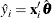RD

specifies a variable to contain the robust MCD Mahalanobis distances. For the definition of robust distance, see the section Robust Distance.

RESIDUAL | R

specifies a variable to contain the unstandardized residuals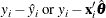SRESIDUAL | SR

specifies a variable to contain the standardized residuals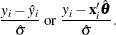By default, the LTS method uses Wscale as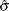for computing the standardized residuals.

STDP

specifies a variable to contain the estimates of the standard errors of the estimated mean responses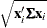where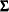denotes the covariance matrix of the parameter estimates. You can request the ODS table of this covariance matrix by specifying the COVB option in the MODEL statement. The STDP= option is applied to M, S, and MM estimation, but not to LTS estimation.

STDI

specifies a variable to contain the estimates of the standard errors of the individual predicted values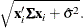The STDI= option is applied to M, S, and MM estimation, but not to LTS estimation.

WEIGHT

specifies a variable to contain the computed final weights.